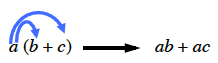### Home > MC2 > Chapter 6 > Lesson 6.1.1 > Problem6-9

6-9.

Use the Distributive Property to simplify the following expressions.

1. $4(x + 2)$

1. $–5(9 + x)$

1. $7(x – 3)$

The distributive property involves multiplying the first number into each
individual value inside the parenthesis and then combining the terms.$−45 − 5x$
Be sure to know how to get this answer.